## 2.2.8 Mechanical Subsystem

This thesis deals with mechanical phenomena mainly caused by electro-thermal stress conditions. Since the electrical burden produces heat and the heat non-negligible volume expansion, the mechanical part has to be considered as well. The basic equation used for TCAD purposes is HOOKE's2.27 law which has been originally introduced by the words Ut tensio sic vis''2.28. The corresponding formula reads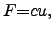(2.107)

where the absolute value of the applied force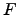to a body is proportional to its elongation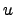. Here the constant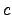determines the stiffness of the body. More generally, HOOKE's law can be formulated for local quantities in a body where the local stress tensor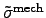is associated to the GREEN2.29 tensor (local strain tensor)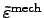for a given body by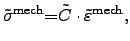(2.108)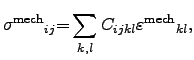(2.109)

where the proportionality factor is determined by the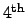-rank stiffness tensor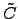and the strain is defined according to CAUCHY2.30via local displacements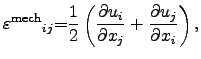(2.110)

where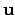is the displacement or deformation vector andthe local position.

Using the VOIGT2.31 notation [96,97,98], the ranks of the tensors involved in (2.108) can be reduced due to the symmetry of the material and due to the symmetry according to energy conservation laws . Thus, the number of independent tensor entities reduces from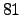to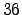by material symmetry and further reduces to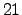mutual independent tensor entities due to energy conservation [96,59]. Therefore, equation (2.108) can be expressed as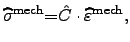(2.111)

where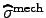andare the vector-valued quantities for the mechanical stress and strain in the VOIGT notation, respectively. Furthermore,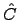represents the stiffness matrix of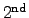rank also in the VOIGT notation.

In TCAD applications of modern devices it is often sufficient to deal with static stresses, only. In that cases, the speed of involved particles can be neglected . The mechanical equations have to fulfill general conservations laws [96,97] for energy, momentum, angular momentum, and mass. Thus, the mechanical subsystem can be described by the local conservation laws of energy, momentum, and mass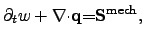(2.112)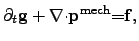(2.113)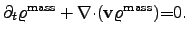(2.114)

In (2.112) the local energy density is denoted by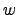, the energy flux density by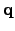, and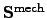represents the mechanical power density. The latter equation represents the mechanical analogon of the POYNTING vector. Equation (2.113) is the momentum conservation equation, where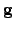is the momentum density,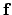is the local force density,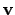the velocity of the moving particles, and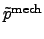is the momentum flux density which is often called pressure tensor. Equation (2.114) presents the local mass continuity equation, where the specific mass density is denoted as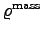. If mass fluxes have to be considered, for instance in electro-migration analysis, the kinetic pressure tensorbecomes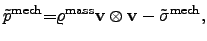(2.115)

where the specific mass density is denoted by,is the stress tensor, andis the speed of the moving particles.

Later on,can be also used as a scalar-valued quantity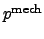when the simplified VOIGT notation is used: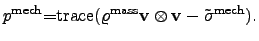(2.116)

If the flux of mass has not to be considered, the associated velocity of the particles becomes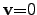and the hydrostatic pressure can be determined by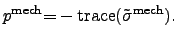(2.117)

The definitions of hydrostatic pressurein (2.116) and (2.117) can be used as a metric which provides a possibility to visualize, to compare within measurements, or to define a figure of merit in an optimization loop .

If moving particles are considered, the mechanical analogon to the electrical continuity equation is the mass continuity equation (2.114) and can be treated with the EULERian2.32 continuity equation(2.118)

which is the mass conservation equation (2.114). A mathematical coupling between the mass flux and the mechanical stress can be obtain by using the first law for continuity mechanics from CAUCHY(2.119)

where therepresents the externally applied force density.

Stefan Holzer 2007-11-19